# #SWDchallenge: Graph makeover

At storytelling with data, they run a monthly challenge on data visualization named #SWDchallenge. The main idea of the challenges is to test data visualization and storytelling skills.

Each month the challenge has a different topic. This month the challenge was to improve an existing graph. I decided to take a previous graph of mine that was published on a paper that was published on 2015.

## The "original"

The following is the graph that we are going to start with.

This is not the exact same graph that was presented in the article on 2015, but it serves the purpose of the challenge. The data can be downloaded from here. This graph present the fraction of energy ($\eta$) transmitted for helical composites with different geometrical parameters. The parameters varied were:

• Pitch angle $\alpha$: the angle between consecutive layers;
• Composite thickness $D$, that is normalized to the wavelength $\lambda$; and
• Number of layers $N$ in each cell of the composite.

The following schematic illustrate these parameters.I would not say that the graph is awful, and, in comparison to what you would find in most scientific literature it is even good. But … in the land of the blind, the one-eyed is king. So, let's enumerate what are the sins of the plot and see if we can correct them:

• It has two x axes.
• The legend is enclosed in a box that seems unnecessary.
• Right and top spines are not contributing to the plot.
• Annotations are stealing protagonism from the data.
• It looks clutterd with lines and markers.
• It is a spaghetti graph.

The following snippet generates this graph.

import numpy as np
from matplotlib import pyplot as plt
from matplotlib import rcParams

rcParams['font.family'] = 'serif'
rcParams['font.size'] = 16
rcParams['legend.fontsize'] = 15
rcParams['mathtext.fontset'] = 'cm'

markers = ['o', '^', 'v', 's', '<', '>', 'd', '*', 'x', 'D', '+', 'H']
usecols=range(1, 9))
labels = labels[0, :]

fig = plt.figure()
ax = plt.subplot(111)
for cont in range(8):
plt.plot(data[:, 0], data[:, cont + 1], marker=markers[cont],
label=r"$D/\lambda={:.3g}$".format(labels[cont]))

# First x-axis
xticks, xlabels = plt.xticks()
plt.xlabel(r"Number of layers - $N$", size=15)
plt.ylabel(r"Fraction of Energy - $\eta$", size=15)
ax.legend(loc='center left', bbox_to_anchor=(1, 0.5))

# Second x-axis
ax2 = ax.twiny()
ax2.set_xticks(xticks[2:])
ax2.set_xticklabels(180./xticks[2:])
plt.xlabel(r"Angle - $\alpha\ (^\circ)$", size=15)

plt.tight_layout()
plt.savefig("energy_vs_D_orig.svg")
plt.savefig("energy_vs_D_orig.png", dpi=300)


## Corrections

I will vindicate the graph one sin at a time, let's see how it turns out.

### It has two x axes

I, originally, added two axes to show both the number of layers and the angle between them at the same time. The general recommendation is to avoid this, so let's get rid of the top one.

### Legend in a box

Pretty straightforward …

### Right and top spines

Let's remove them

### Annotations are stealing protagonism

Let's move all the annotations to the background by changing the color to a lighter gray.

### Clutterd with lines and markers

Let's just keep the lines.

And increase the linewidth.

### It is a spaghetti graph

I think that a good option for this graph would be to highlight one line at a time. Doing this, we end up with.

The following snippet generates this version.

import numpy as np
from matplotlib import pyplot as plt
from matplotlib import rcParams

# Plots setup
gray = '#757575'
plt.rcParams["mathtext.fontset"] = "cm"
plt.rcParams["text.color"] = gray
plt.rcParams["xtick.color"] = gray
plt.rcParams["ytick.color"] = gray
plt.rcParams["axes.labelcolor"] = gray
plt.rcParams["axes.edgecolor"] = gray
plt.rcParams["axes.spines.right"] = False
plt.rcParams["axes.spines.top"] = False
rcParams['font.family'] = 'serif'
rcParams['mathtext.fontset'] = 'cm'

def line_plots(data, highlight_line, title):
plt.title(title)
for cont, datum in enumerate(data[:, 1:].T):
if cont == highlight_line:
plt.plot(data[:, 0], datum, zorder=3, color="#984ea3",
linewidth=2)
else:
plt.plot(data[:, 0], datum, zorder=2, color=gray,
linewidth=1, alpha=0.5)

usecols=range(1, 9))
labels = labels[0, :]

plt.close("all")
plt.figure(figsize=(8, 4))
for cont in range(8):
ax = plt.subplot(2, 4, cont + 1)
title = r"$D/\lambda={:.3g}$".format(labels[cont])
line_plots(data, cont, title)
plt.ylim(0.4, 1.0)
if cont < 4:
plt.xlabel("")
ax.xaxis.set_ticks([])
ax.spines["bottom"].set_color("none")
else:
plt.xlabel(r"Number of layers - $N$")
if cont % 4 > 0:
ax.yaxis.set_ticks([])
ax.spines["left"].set_color("none")
else:
plt.ylabel(r"Fraction of Energy - $\eta$")

plt.tight_layout()
plt.savefig("energy_vs_D_7.svg")
plt.savefig("energy_vs_D_7.png", dpi=300)


## Final tweaks

Using Inkscape I added some final details to get the following version.

# Isometric graphics in Inkscape: Part 2

Last week I posted a quick guide on isometric drawing using Inkscape. In that post I showed how to obtain images that are projected to the faces of an isometric box.

After my post, I was asked by Biswajit Banerjee on Twitter if I could repeat the process with a more complex example, and he suggested the following schematicwhich, I guess, was created in Inkscape using the "Create 3D Box" option.

In this post, I will:

1. Use the same approach from last week to recreate this schematic
2. Suggest my preferred approach for drawing this schematic

## First approach

I will repeat the cheatsheet from last week. Keep in mind that Inkscape treats clockwise rotation as positive. Opposite to common notation in mathematics.

Then, to create a box with desired dimensions we first create each rectangle with the right dimensiones (in parallel projections). In the following example we used faces with aspect ratios 3:2, 2:1 and 4:3. The box at the right is the figure obtained after applying the transformations described in the previous schematic.

We can now proceed, to recreate the desired figure. I don't know the exact dimensions of the box; my guess is that the cross section was a square and the aspect ratio was 5:1. After applying the transformations to each rectangle we obtain the following (adding some tweaks here and there).

## Second approach

For this type of schematic I would prefer to create an axonometric grid (File > Document Properties > Grids). After adding the grid to our canvas it is pretty straightforward to draw the figures in isometric view. The canvas should look similar to the following image.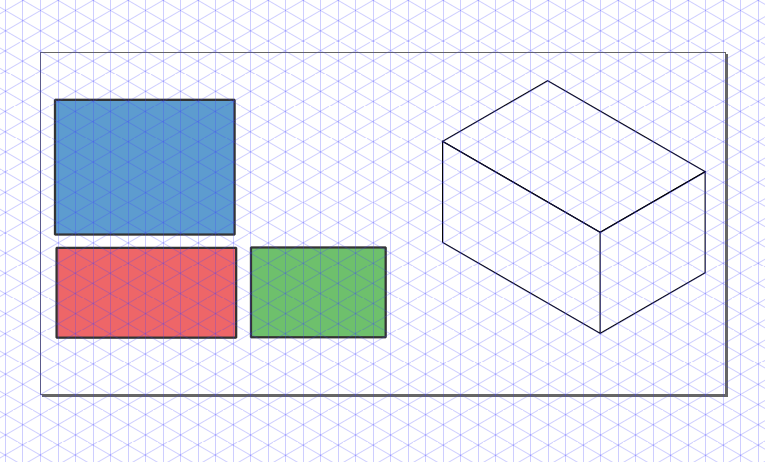We can then draw each face using the grid. If we want to be more precise we can activate Snap to Cusp Nodes. The following animation shows the step by step construction.And we obtain the final image.

## Conclusion

As I mentioned, Inkscape can be used for drawing simple figures in isometric projection. Nevertheless, I strongly suggest to use a CAD like FreeCAD for more compicated geometries.

# Isometric graphics in Inkscape

Sometimes I find myself in need of making a schematic using an isometric projection. When the schematic is complicated the best shot is to use some CAD like FreeCAD, but sometimes it's just needed in simple diagrams. Another situation where this is a common needed is in video games, where "isometric art" and pixel art are pretty common.

What we want is depicted in the following figure.

That is, we want to start with some information that is drawn, or written in the case of the example, and we want to obtain how would it been seen on one of the faces of an isometric box.

Following, I will describe briefly the transformations involved in this process. If you are just interested in the recipe for doing this in Inkscape, skip to the end of this post.

## Transformations involved

Since we are working on a computer screen, we are talking of 2 dimensions. Hence, all transformations are represented by 2×2 matrices. In the particular example of interest in this post we need the following transformations:

1. Vertical scaling
2. Horizontal skew
3. Rotation

Following are the transformation matrices.

### Scaling in the vertical direction

The matrix is given by

\begin{equation*} M_\text{scaling} = \begin{bmatrix} 1 &0\\ 0 &a\end{bmatrix}\, , \end{equation*}

where $a$ is the scaling factor.

### Horizontal skew

The matrix is given by

\begin{equation*} M_\text{skew} = \begin{bmatrix} 1 &\tan a\\ 0 &1\end{bmatrix}\, , \end{equation*}

where $a$ is the skewing angle.

### Rotation

The matrix is given by

\begin{equation*} M_\text{rotation} = \begin{bmatrix} \cos a &-\sin a\\ \sin a &\cos a\end{bmatrix}\, , \end{equation*}

where $a$ is the rotation angle.

### Complete transformation

The complete transformation is given by

\begin{equation*} M_\text{complete} = M_\text{rotation} M_\text{skew} M_\text{scaling}\, , \end{equation*}

particularly,

\begin{align*} &M_\text{side} = \frac{1}{2}\begin{bmatrix} \sqrt{3} & 0\\ -1 &2\end{bmatrix}\approx \begin{bmatrix} 0.866 & 0.000\\ -0.500 &1.000\end{bmatrix}\, , \\ &M_\text{front} = \frac{1}{2}\begin{bmatrix} \sqrt{3} & 0\\ 1 &2\end{bmatrix}\approx \begin{bmatrix} 0.866 & 0.000\\ 0.500 &1.000\end{bmatrix}\, , \\ &M_\text{plant} = \frac{1}{2}\begin{bmatrix} \sqrt{3} & -\sqrt{3}\\ -1 &1\end{bmatrix}\approx \begin{bmatrix} 0.866 & -0.866\\ 0.500 &0.500\end{bmatrix}\, . \end{align*}

The values seem a bit arbitrary, but they can be obtained from the isometric projection itself. But that explanation would be a bit too long for this post.

### Tranformation in Inkscape

We already have the transformation matrices and we can use them in Inkscape. But first, we need to understand how it works. Inkscape, uses SVG, the web standard for vector graphics. Transformations in SVG are done using the following matrix

\begin{equation*} \begin{bmatrix} a &c &e\\ b &d &f\\ 0 &0 &1\end{bmatrix}\, , \end{equation*}

that uses homogeneous coordinates. So, one can just press Shift + Ctrl + M and type the components of the matrices obtaines above for $a$, $b$, $c$, and $d$; leaving $e$ and $f$ as zero.

My preferred method, though, is to apply each transformation after the other in the Transform dialog (Shift + Ctrl + M). And, this is the method presented in the cheatsheet at the bottom of this post.

## TL;DR

If you are just interested in the transformations needed in Inkscape you can check the cheatsheet below. It uses third-angle as presented below.

### Cheatsheet

Keep in mind that Inkscape treats clockwise rotation as positive. Opposite to common notation in mathematics.

# Finite differences in infinite domains

Because of my friend, Edward Villegas, I ended up thinking about using a change of variables when solving an eigenvalue problem with finite difference.

## The problem

Let's say that we want to solve a differential equation over an infinite domain. A common case is to solve the Time independent Schrödinger equation subject to a potential $V(x)$. For example

\begin{equation*} -\frac{1}{2}\frac{\mathrm{d}^2}{\mathrm{d}x^2}\psi(x) + V(x) \psi(x) = E\psi(x),\quad \forall x\in (-\infty, \infty), \end{equation*}

where we want to find the pairs of eigenvalues/eigenfunctions $(E_n, \psi_n(x))$.

What I normally do when using finite differences is to regularly divide the domain. Where I take a large enough domain, so the solution have decayed close to zero. What I do in this post is to make a change of variable to render the interval finite first and then regularly divide the transformed domain in finite intervals.

## My usual approach

My usual approach is to approach the second derivative with a centered difference for the point $x_i$ like this

\begin{equation*} \frac{\mathrm{d}^2 f(x)}{\mathrm{d}x^2} \approx \frac{f(x + \Delta x) - 2 f(x_i) + f(x - \Delta x)}{\Delta x^2}\, , \end{equation*}

with $\Delta x$ the separation between points.

We can solve this in Python with the following snippet:

import numpy as np
from scipy.sparse import diags
from scipy.sparse.linalg import eigs

def regular_FD(pot, npts=101, x_max=10, nvals=6):
"""
Find eigenvalues/eigenfunctions for Schrodinger
equation for the given potential pot using
finite differences
"""
x = np.linspace(-x_max, x_max, npts)
dx = x - x
D2 = diags([1, -2, 1], [-1, 0, 1], shape=(npts, npts))/dx**2
V = diags(pot(x))
H = -0.5*D2 + V
vals, vecs = eigs(H, k=nvals, which="SM")
return x, np.real(vals), vecs


Let's setup the plotting details.

# Jupyter notebook plotting setup & imports
%matplotlib notebook
import matplotlib.pyplot as plt

gray = '#757575'
plt.rcParams["figure.figsize"] = 6, 4
plt.rcParams["mathtext.fontset"] = "cm"
plt.rcParams["text.color"] = gray
fontsize = plt.rcParams["font.size"] = 12
plt.rcParams["xtick.color"] = gray
plt.rcParams["ytick.color"] = gray
plt.rcParams["axes.labelcolor"] = gray
plt.rcParams["axes.edgecolor"] = gray
plt.rcParams["axes.spines.right"] = False
plt.rcParams["axes.spines.top"] = False


Let's consider the Quantum harmonic oscillator, that has as eigenvalues

\begin{equation*} E_n = n + \frac{1}{2},\quad \forall n = 0, 1, 2 \cdots \end{equation*}

Using the finite difference method we have values that are really close to the analytic ones.

x, vals, vecs = regular_FD(lambda x: 0.5*x**2, npts=201)
vals


with output

array([0.4996873 , 1.49843574, 2.49593063, 3.49216962, 4.48715031,
5.4808703 ])


With the analytic ones

[0.5, 1.5, 2.5, 3.5, 4.5, 5.5])


If we plot these two, we obtain the following.

plt.figure()
plt.plot(anal_vals)
plt.plot(vals, "o")
plt.xlabel(r"$n$", fontsize=16)
plt.ylabel(r"$E_n$", fontsize=16)
plt.legend(["Analytic", "Finite differences"])
plt.tight_layout();


Let's see the eigenfunctions.

plt.figure()
plt.plot(x, np.abs(vecs[:, :3])**2)
plt.xlim(-6, 6)
plt.xlabel(r"$x$", fontsize=16)
plt.ylabel(r"$|\psi_n(x)|^2$", fontsize=16)
plt.yticks([])
plt.tight_layout();


One inconvenient with this method is the redundant sampling towards the extreme of the intervals, while under sample the middle part.

## Changing the domain

Let's now consider the case where we transform the infinite domain to a finite one with a change of variable

\begin{equation*} \xi = \xi(x) \end{equation*}

with $\xi \in (-1, 1)$. Two options for this transformation are:

• $\xi = \tanh x$; and
• $\xi = \frac{2}{\pi} \arctan x$.

Making this change of variable the equation we need to solve the following equation

\begin{equation*} -\frac{1}{2}\left(\frac{\mathrm{d}\xi}{\mathrm{d}x}\right)^2\frac{\mathrm{d}^2}{\mathrm{d}\xi^2}\psi(\xi) - \frac{1}{2}\frac{\mathrm{d}^2\xi}{\mathrm{d}x^2}\frac{\mathrm{d}}{\mathrm{d}\xi}\psi(\xi) + V(\xi) \psi(\xi) = E\psi(\xi) \end{equation*}

The following snippet solve the eigenproblem for the mapped domain:

def mapped_FD(pot, fun, dxdxi, dxdxi2, npts=101, nvals=6, xi_tol=1e-6):
"""
Find eigenvalues/eigenfunctions for Schrodinger
equation for the given potential pot using
finite differences over a mapped domain on (-1, 1)
"""
xi = np.linspace(-1 + xi_tol, 1 - xi_tol, npts)
x = fun(xi)
dxi = xi - xi
D2 = diags([1, -2, 1], [-1, 0, 1], shape=(npts, npts))/dxi**2
D1 = 0.5*diags([-1, 1], [-1, 1], shape=(npts, npts))/dxi
V = diags(pot(x))
fac1 = diags(dxdxi(xi)**2)
fac2 = diags(dxdxi2(xi))
H = -0.5*fac1.dot(D2) - 0.5*fac2.dot(D1) + V
vals, vecs = eigs(H, k=nvals, which="SM")
return x, np.real(vals), vecs


### First transformation: $\xi = \tanh(x)$

Let's consider first the transformation

\begin{equation*} \xi = \tanh(x)\, . \end{equation*}

In this case

\begin{equation*} \frac{\mathrm{d}\xi}{\mathrm{d}x} = 1 - \tanh^2(x) = 1 - \xi^2\, , \end{equation*}

and

\begin{equation*} \frac{\mathrm{d}^2\xi}{\mathrm{d}x^2} = -2\tanh(x)[1 - \tanh^2(x)] = -2\xi[1 - \xi^2]\, . \end{equation*}

We need to define the functions

pot = lambda x: 0.5*x**2
fun = lambda xi: np.arctanh(xi)
dxdxi = lambda xi: 1 - xi**2
dxdxi2 = lambda xi: -2*xi*(1 - xi**2)


and run the function

x, vals, vecs = mapped_FD(pot, fun, dxdxi, dxdxi2, npts=201)
vals


And we obtain the following

array([0.49989989, 1.4984226 , 2.49003572, 3.46934257, 4.46935021,
5.59552989])


If we compare with the analytic values we get the following.

plt.figure()
plt.plot(anal_vals)
plt.plot(vals, "o")
plt.legend(["Analytic", "Finite differences"])
plt.xlabel(r"$n$", fontsize=16)
plt.ylabel(r"$E_n$", fontsize=16)
plt.tight_layout();


And the following are the eigenfunctions.

plt.figure()
plt.plot(x, np.abs(vecs[:, :3])**2)
plt.xlim(-6, 6)
plt.xlabel(r"$x$", fontsize=16)
plt.ylabel(r"$|\psi_n(x)|^2$", fontsize=16)
plt.yticks([])
plt.tight_layout();


### Second transformation: $\xi = \frac{2}{\pi}\mathrm{atan}(x)$

Let's consider first the transformation

\begin{equation*} \xi = \frac{2}{\pi}\mathrm{atan}(x)\, . \end{equation*}

In this case

\begin{equation*} \frac{\mathrm{d}\xi}{\mathrm{d}x} = \frac{2}{\pi(1 + x^2)} = \frac{2 \cos^2\xi}{\pi} \, , \end{equation*}

and

\begin{equation*} \frac{\mathrm{d}^2\xi}{\mathrm{d}x^2} = -\frac{4x}{\pi(1 + x^2)^2} = -\frac{4 \cos^4\xi \tan \xi}{\pi}\, . \end{equation*}

Once, again, we define the functions.

pot = lambda x: 0.5*x**2
fun = lambda xi: np.tan(0.5*np.pi*xi)
dxdxi = lambda xi: 2/np.pi * np.cos(0.5*np.pi*xi)**2
dxdxi2 = lambda xi: -4/np.pi * np.cos(0.5*np.pi*xi)**4 * np.tan(0.5*np.pi*xi)


and run the function

x, vals, vecs = mapped_FD(pot, fun, dxdxi, dxdxi2, npts=201)
vals


to obtain the following eigenvalues

array([0.49997815, 1.49979632, 2.49930872, 3.49824697, 4.49627555,
5.49295665])


with this plot

plt.figure()
plt.plot(anal_vals)
plt.plot(vals, "o")
plt.legend(["Analytic", "Finite differences"])
plt.xlabel(r"$n$", fontsize=16)
plt.ylabel(r"$E_n$", fontsize=16)
plt.tight_layout();


and the following eigenfunctions.

plt.figure()
plt.plot(x, np.abs(vecs[:, :3])**2)
plt.xlabel(r"$x$", fontsize=16)
plt.ylabel(r"$|\psi|^2$", fontsize=16)
plt.xlim(-6, 6)
plt.xlabel(r"$x$", fontsize=16)
plt.ylabel(r"$|\psi_n(x)|^2$", fontsize=16)
plt.yticks([])
plt.tight_layout();


## Conclusion

The method works fine, although the differential equation is more convoluted due to the change of variable. Although there are more elegant methods to consider infinite domains, this is simple enough to be solved in 10 lines of code.

We can see that the mapping $\xi = \mathrm{atan}(x)$, covers better the domain than $\xi = \tanh(x)$, where most of the points are placed in the center of the interval.

This post was written in the Jupyter notebook. You can download this notebook, or see a static view on nbviewer.

# Cyclic colormaps comparison

I started this post looking for a diffusion map on Python, that I didn't find. Then I continued following an example on manifold learning by Jake Vanderplas on a different dataset. It worked nicely,

but the colormap used is Spectral, that is divergent. This made me think about using a cyclic colormap, and ended up in this StackOverflow question. And I decided to compare some cyclic colormaps.

I picked up colormaps from different sources

• cmocean:
• phase
• Paraview:
• hue_L60
• erdc_iceFire
• nic_Edge
• Colorcet:
• colorwheel
• cyclic_mrybm_35_75_c68
• cyclic_mygbm_30_95_c78

and, of course, hsv. You can download the colormaps in text format from here.

## Comparison

For all the examples below the following imports are done:

from __future__ import division, print_function
import numpy as np
import matplotlib.pyplot as plt
from matplotlib.colors import LinearSegmentedColormap
from colorspacious import cspace_convert


### Color map test image

Peter Kovesi proposed a way to compare cyclic colormaps on a paper on 2015. It consists of a spiral ramp with an undulation. In polar coordinates it reads

\begin{equation*} c = (A \rho^p \sin(n \theta) + \theta)\, \mathrm{mod}\, 2\pi \end{equation*}

with $A$ the amplitude of the oscilation, $\rho$ the normalized radius in [0, 1], $p$ a positive number, and $n$ the number of cycles.

And the following function creates the grid in Python

def circle_sine_ramp(r_max=1, r_min=0.3, amp=np.pi/5, cycles=50,
power=2, nr=50, ntheta=1025):
r, t = np.mgrid[r_min:r_max:nr*1j, 0:2*np.pi:ntheta*1j]
r_norm = (r - r_min)/(r_max - r_min)
vals = amp * r_norm**power * np.sin(cycles*t) + t
vals = np.mod(vals, 2*np.pi)
return t, r, vals


The following is the result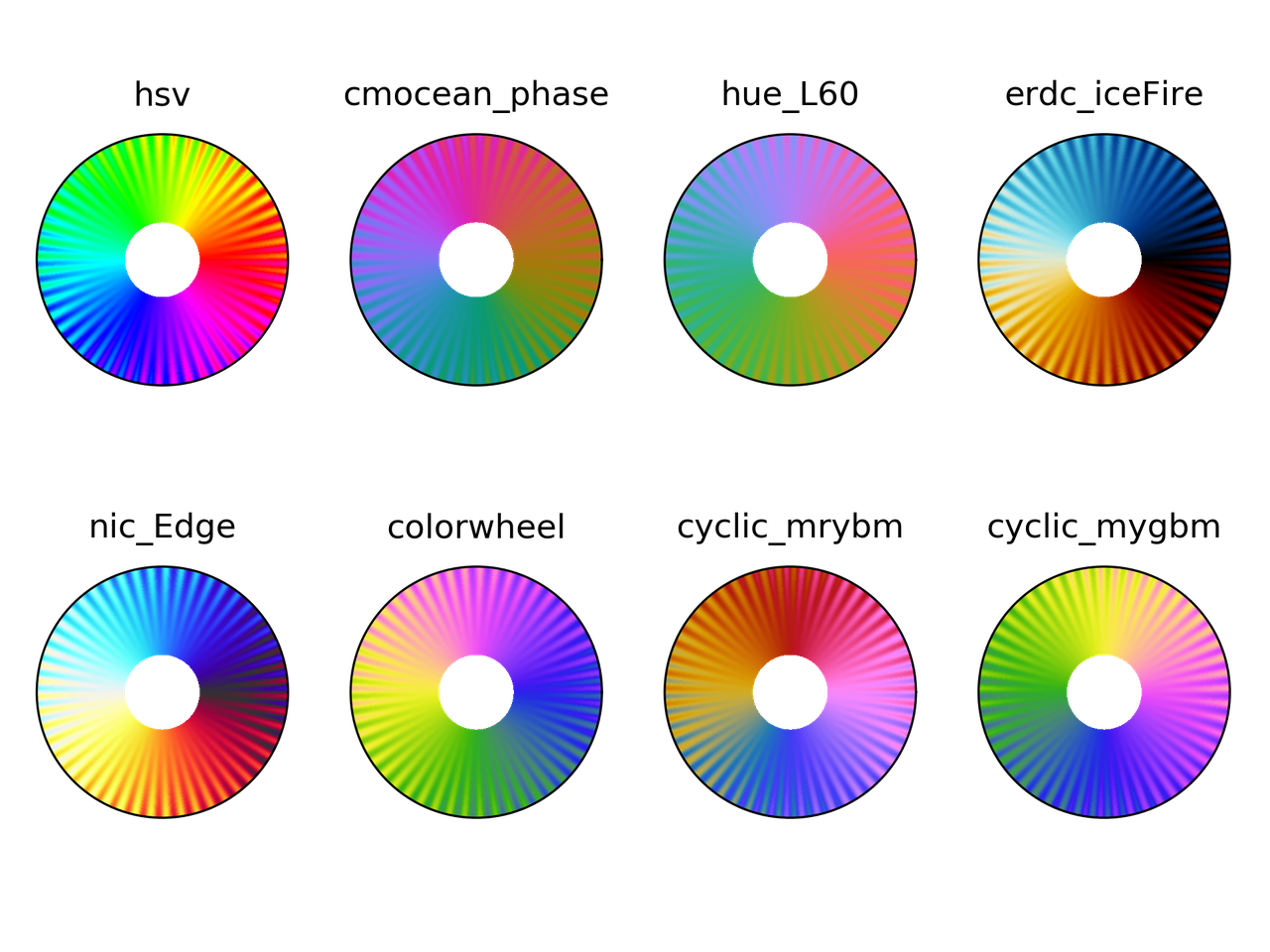### Colorblindness test

t, r, vals = circle_sine_ramp(cycles=0)
cmaps = ["hsv",
"cmocean_phase",
"hue_L60",
"erdc_iceFire",
"nic_Edge",
"colorwheel",
"cyclic_mrybm",
"cyclic_mygbm"]
severity = [0, 50, 50, 50]
for colormap in cmaps:
fig = plt.figure()
for cont in range(4):
cvd_space = {"name": "sRGB1+CVD",
"cvd_type": cvd_type[cont],
"severity": severity[cont]}
data2 = cspace_convert(data, cvd_space, "sRGB1")
data2 = np.clip(data2, 0, 1)
cmap = LinearSegmentedColormap.from_list('my_colormap', data2)
ax = plt.subplot(2, 2, 1 + cont, projection="polar")
ax.pcolormesh(t, r, vals, cmap=cmap)
ax.set_xticks([])
ax.set_yticks([])
plt.suptitle(colormap)
plt.tight_layout()
plt.savefig(colormap + ".png", dpi=300)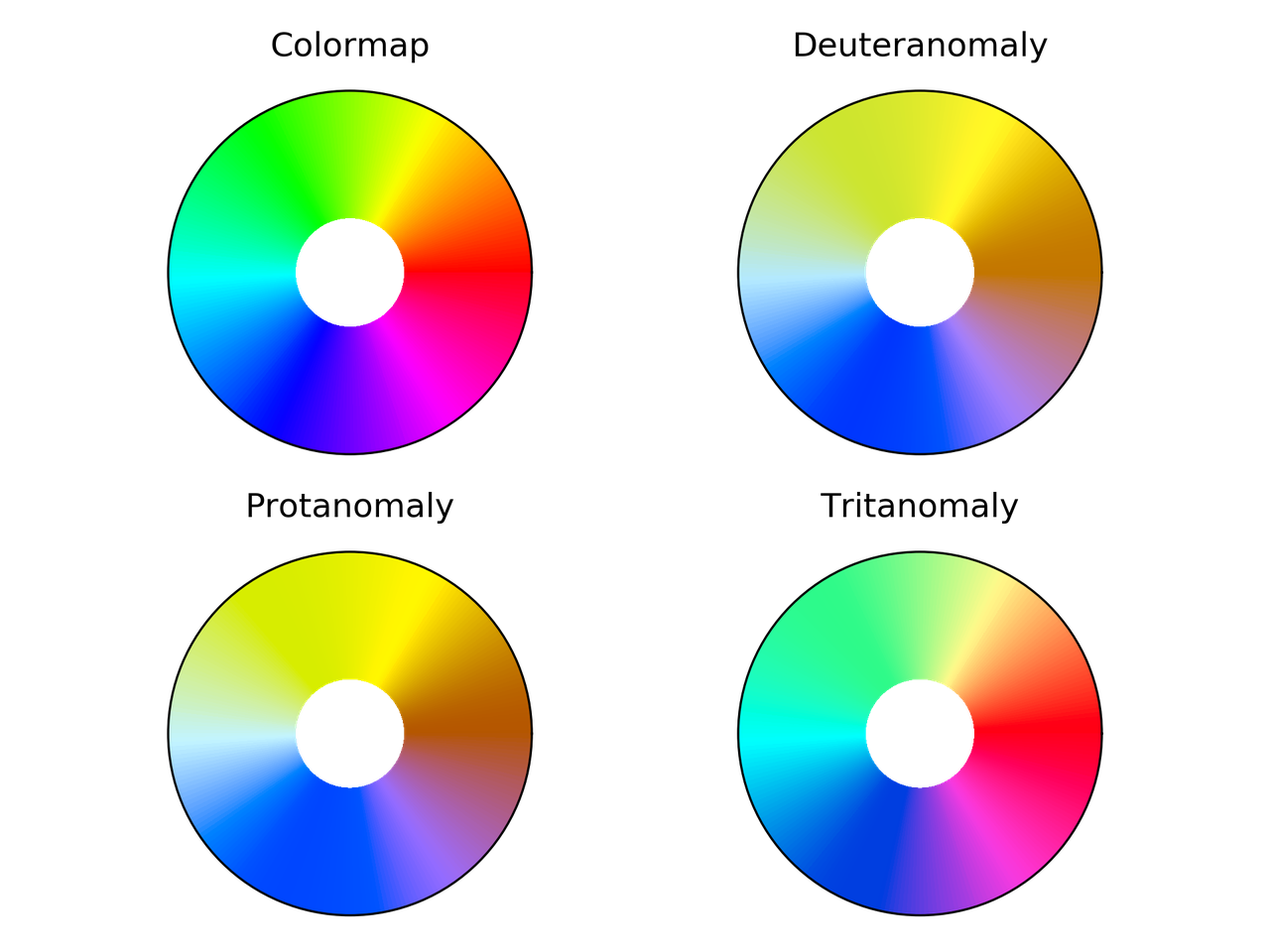hsv colormap comparison for different color vision deficiencies.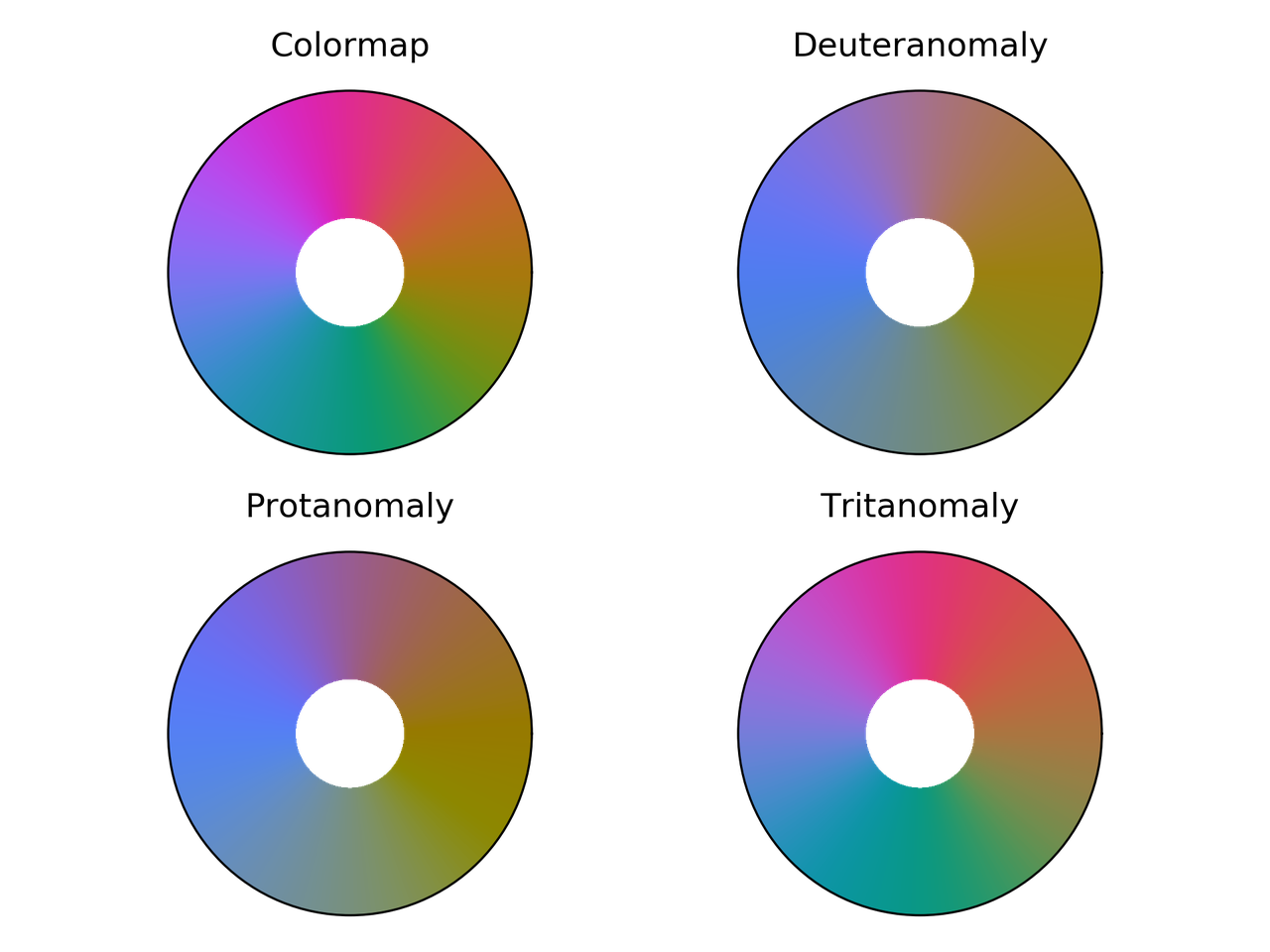Phase colormap comparison for different color vision deficiencies.hue_L60 colormap comparison for different color vision deficiencies.erdc_iceFire colormap comparison for different color vision deficiencies.nic_Edge colormap comparison for different color vision deficiencies.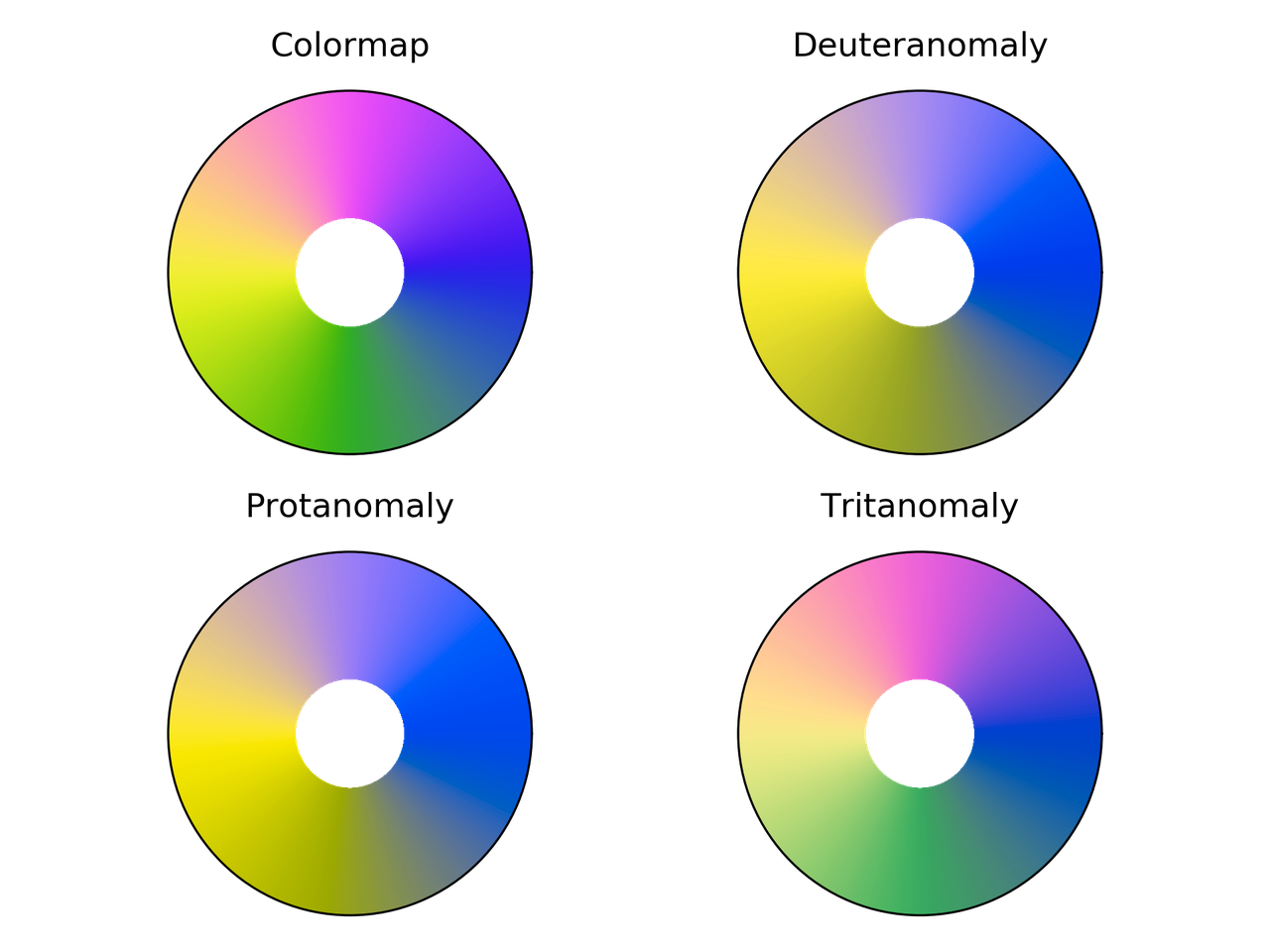Colorwheel colormap comparison for different color vision deficiencies.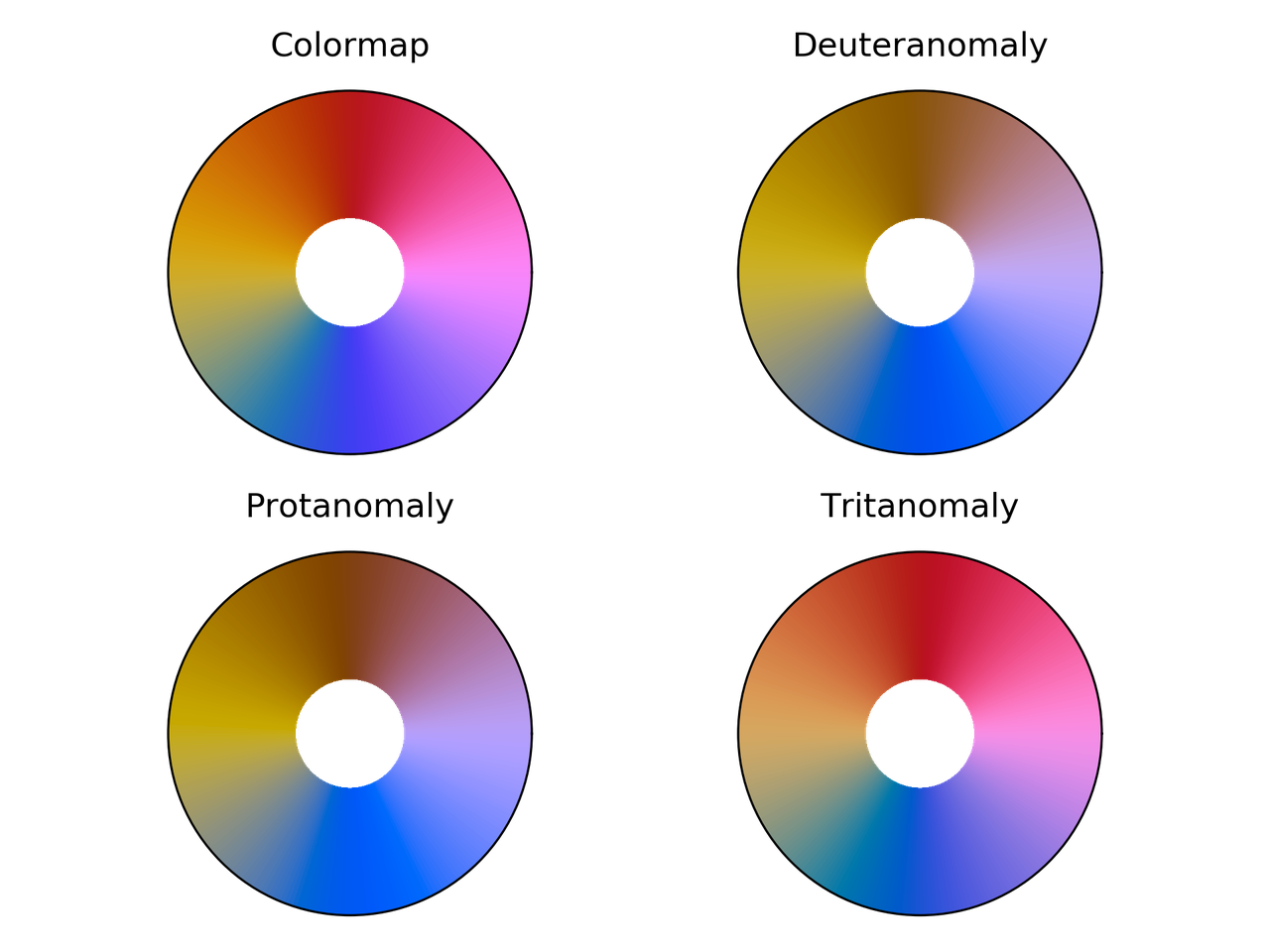Cyclic_mrybm colormap comparison for different color vision deficiencies.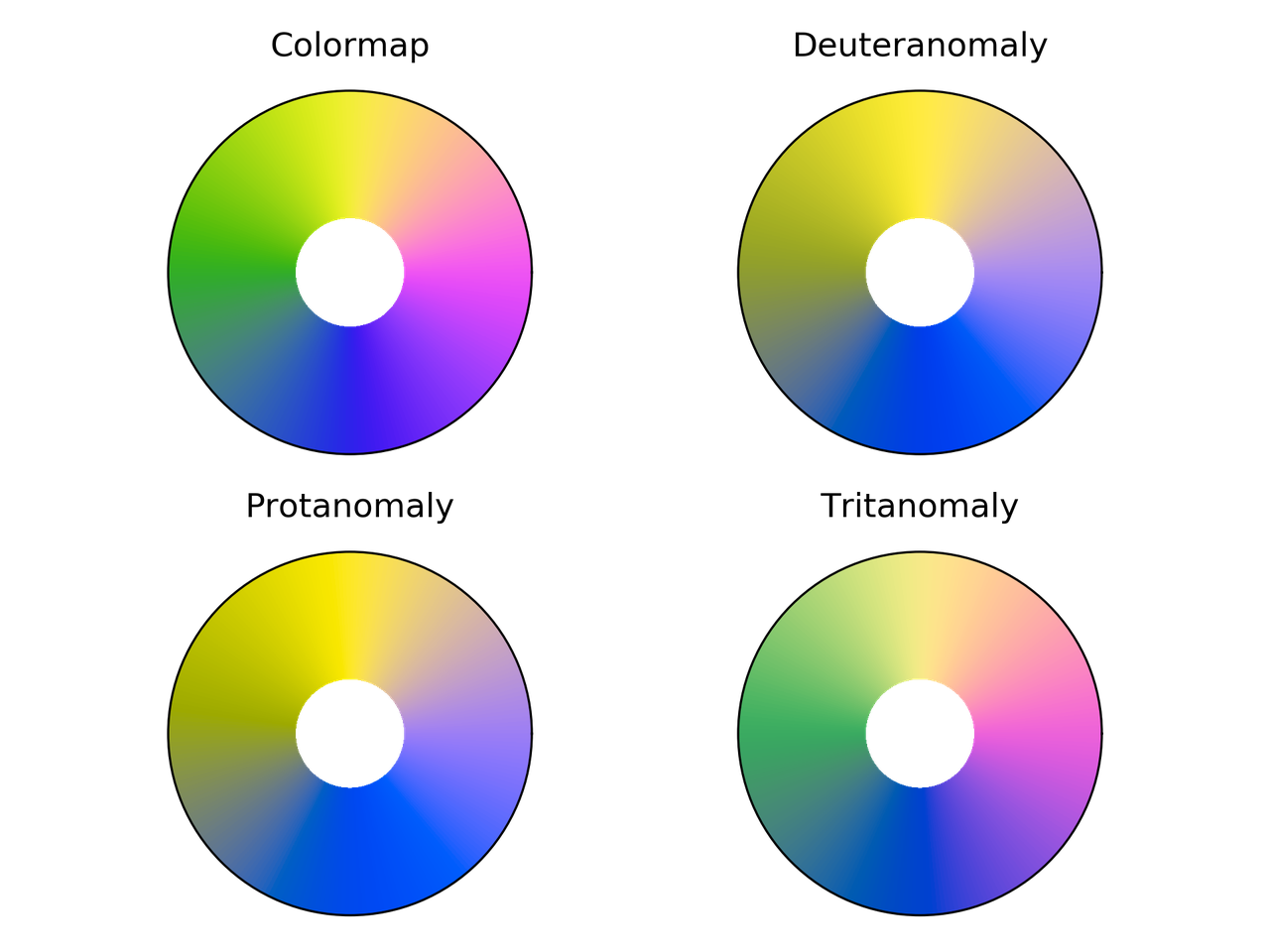Cyclic_mygbm colormap comparison for different color vision deficiencies.

## Randomly generated cyclic colormaps

What if we generate some random colormaps that are cyclic? How would they look like?

Following are the snippet and resulting colormaps.

from __future__ import division, print_function
import numpy as np
from mpl_toolkits.mplot3d import Axes3D
import matplotlib.pyplot as plt
from matplotlib.colors import LinearSegmentedColormap

# Next line to silence pyflakes. This import is needed.
Axes3D

plt.close("all")
fig = plt.figure()
fig2 = plt.figure()
nx = 4
ny = 4
azimuths = np.arange(0, 361, 1)
zeniths = np.arange(30, 70, 1)
values = azimuths * np.ones((30, 361))
for cont in range(nx * ny):
np.random.seed(seed=cont)
mat = np.random.rand(3, 3)
rot_mat, _ = np.linalg.qr(mat)
t = np.linspace(0, 2*np.pi, 256)
z = 0.0*np.cos(t)
X = np.vstack((x, y, z))
X = rot_mat.dot(X) + center

ax = fig.add_subplot(ny, nx, 1 + cont, projection='polar')
cmap = LinearSegmentedColormap.from_list('my_colormap', X.T)
ax.pcolormesh(azimuths*np.pi/180.0, zeniths, values, cmap=cmap)
ax.set_xticks([])
ax.set_yticks([])

ax2 = fig2.add_subplot(ny, nx, 1 + cont, projection='3d')
ax2.plot(X[0, :], X[1, :], X[2, :])
ax2.set_xlim(0, 1)
ax2.set_ylim(0, 1)
ax2.set_zlim(0, 1)
ax2.view_init(30, -60)
ax2.set_xticks([0, 0.5, 1.0])
ax2.set_yticks([0, 0.5, 1.0])
ax2.set_zticks([0, 0.5, 1.0])
ax2.set_xticklabels([])
ax2.set_yticklabels([])
ax2.set_zticklabels([])

fig.savefig("random_cmaps.png", dpi=300, transparent=True)
fig2.savefig("random_cmaps_traj.svg", transparent=True)16 randomly generated colormaps.

Trajectories in RGB space for the randomly generated colormaps.

A good idea would be to take these colormaps and optimize some perceptual parameters such as lightness to get some usable ones.

## Conclusions

I probably will use phase, colorwheel, or mrybm in the future.

Initial image using phase.

Initial image using colorwheel.

Initial image using mrybm.

# Probability that a random tetrahedron over a sphere contains its center

I got interested in this problem watching the YouTube channel 3Blue1Brown, by Grant Sanderson, where he explains a way to tackle the problem that is just … elegant!

I can't emphasize enough how much I like this channel. For example, his approach to linear algebra in Essence of linear algebra is really good. I mention it, just in case you don't know it.

## The problem

Let's talk business now. The problem was originally part of the 53rd Putnam competition on 1992 and was stated as

Four points are chosen at random on the surface of a sphere. What is the probability that the center of the sphere lies inside the tetrahedron whose vertices are at the four points? (It is understood that each point is in- dependently chosen relative to a uniform distribution on the sphere.)

As shown in the mentioned video, the probability is $1/8$. Let's come with an algorithm to obtain this result —approximately, at least.

## The proposed approach

The approach that we are going to use is pretty straightforward. We are going to obtain a sample of (independent) random sets, with four points each, and check how many of them satisfy the condition of being inside the tetrahedron with the points as vertices.

For this approach to work, we need two things:

1. A way to generate random numbers uniformly distributed. This is already in numpy.random.uniform, so we don't need to do much about it.
2. A way to check if a point is inside a tetrahedron.

### Checking that a point is inside a tetrahedron

To find if a point is inside a tetrahedron, we could compute the barycentric coordinates for that point and check that all of them are have the same sign. Equivalently, as proposed here, we can check that the determinants of the matrices

\begin{equation*} M_0 = \begin{bmatrix} x_0 &y_0 &z_0 &1\\ x_1 &y_1 &z_1 &1\\ x_2 &y_2 &z_2 &1\\ x_3 &y_3 &z_3 &1 \end{bmatrix}\, , \end{equation*}
\begin{equation*} M_1 = \begin{bmatrix} x &y &z &1\\ x_1 &y_1 &z_1 &1\\ x_2 &y_2 &z_2 &1\\ x_3 &y_3 &z_3 &1 \end{bmatrix}\, , \end{equation*}
\begin{equation*} M_2 = \begin{bmatrix} x_0 &y_0 &z_0 &1\\ x &y & &1\\ x_2 &y_2 &z_2 &1\\ x_3 &y_3 &z_3 &1 \end{bmatrix}\, , \end{equation*}
\begin{equation*} M_3 = \begin{bmatrix} x_0 &y_0 &z_0 &1\\ x_1 &y_1 &z_1 &1\\ x &y &z &1\\ x_3 &y_3 &z_3 &1 \end{bmatrix}\, , \end{equation*}
\begin{equation*} M_4 = \begin{bmatrix} x_0 &y_0 &z_0 &1\\ x_1 &y_1 &z_1 &1\\ x_2 &y_2 &z_2 &1\\ x &y &z &1 \end{bmatrix}\, , \end{equation*}

have the same sign. In this case $(x, y, z)$ is the point of interest and $(x_i, y_i, z_i)$ are the coordinates of each vertex.

## The algorithm

Below is a Python implementation of the approach discussed before

from __future__ import division, print_function
from numpy import (random, pi, cos, sin, sign, hstack,
column_stack, logspace)
from numpy.linalg import det
import matplotlib.pyplot as plt

def in_tet(x, y, z, pt):
"""
Determine if the point pt is inside the
tetrahedron with vertices coordinates x, y, z
"""
mat0 = column_stack((x, y, z, [1, 1, 1, 1]))
det0 = det(mat0)
for cont in range(4):
mat = mat0.copy()
mat[cont] = hstack((pt, 1))
if sign(det(mat)*det0) < 0:
inside = False
break
else:
inside = True
return inside

#%% Computation
prob = []
random.seed(seed=2)
N_min = 1
N_max = 5
N_vals = logspace(N_min, N_max, 100, dtype=int)
for N in N_vals:
inside_cont = 0
for cont_pts in range(N):
phi = random.uniform(low=0.0, high=2*pi, size=4)
theta = random.uniform(low=0.0, high=pi, size=4)
x = sin(theta)*cos(phi)
y = sin(theta)*sin(phi)
z = cos(theta)
if in_tet(x, y, z, [0, 0, 0]):
inside_cont += 1

prob.append(inside_cont/N)

#%% Plotting
plt.figure(figsize=(4, 3))
plt.hlines(0.125, 10**N_min, 10**N_max, color="#3f3f3f")
plt.semilogx(N_vals, prob, "o", alpha=0.5)
plt.xlabel("Number of trials")
plt.ylabel("Computed probability")
plt.tight_layout()
plt.show()


As expected, when the number of samples is sufficiently large, the estimated probability is close to the theoretical value: 0.125. This can be seen in the following figure.

# Numerical methods challenge: Summary

During October (2017) I wrote a program per day for some well-known numerical methods in both Python and Julia. It was intended to be an exercise, then don't expect the code to be good enough for real use. Also, I should mention that I had almost no experience with Julia, so it probably is not idiomatic Julia but more Python-like Julia.

## Summary

This post is a summary of that challenge. For the source code you can check the repository.

### The verdict

Since the challenge is with myself, and the main purpose was to learn some Julia the verdict is: success. Nevertheless, I failed during day 26 with the Boundary Element Method.

### The list of methods

Day Numerical method
01 Bisection
02 Regula falsi
03 Newton
04 Newton multivariate
05 Broyden
08 Newton for optimization
09 Lagrange interpolation
10 Lagrange interpolation with Lobatto sampling
11 Lagrange interpolation using Vandermonde matrix
12 Hermite interpolation
13 Spline interpolation
17 Euler integration
18 Runge-Kutta integration
19 Verlet integration
20 Shooting method
21 Finite differences with Jacobi method
22 Finite differences for eigenvalues
23 Ritz method
24 Finite element method in 1D
25 Finite element method in 2D
26 Boundary element method
27 Monte-Carlo integration
28 LU factorization
29 Cholesky factorization
31 Finite element method with solver

### Conclusions

• This was an exercise of code-kata to learn some of the details of Julia for scientific computing. As such, it was really useful for me to get my hands dirty with Julia.
• Implementing the Boundary Element Method in one day seems to be rough. I knew this beforehand, but I gave it a try anyways ... without succcess.
• Julia syntax is somewhat in a sweetspot between Python and MATLAB. This makes it really easy to use, although the documentation of some packages is at an arcane stage right now.
• I won't take a challenge like this in a while. It takes a lot of atttention to get it done.

# Numerical methods challenge: Day 31

During October (2017) I wrote a program per day for some well-known numerical methods in both Python and Julia. It was intended to be an exercise, then don't expect the code to be good enough for real use. Also, I should mention that I had almost no experience with Julia, so it probably is not idiomatic Julia but more Python-like Julia.

## Putting some things together

Today, I am putting some things together, namely, I am going to solve the system of equations that results in the finite element using the conjugate gradient.

Following are the codes

### Python

from __future__ import division, print_function
import numpy as np
import matplotlib.pyplot as plt

def FEM1D(coords, source):
N = len(coords)
stiff_loc = np.array([[2.0, -2.0], [-2.0, 2.0]])
eles = [np.array([cont, cont + 1]) for cont in range(0, N - 1)]
stiff = np.zeros((N, N))
rhs = np.zeros(N)
for ele in eles:
jaco = coords[ele] - coords[ele]
rhs[ele] = rhs[ele] + jaco*source(coords[ele])
for cont1, row in enumerate(ele):
for cont2, col in enumerate(ele):
stiff[row, col] = stiff[row, col] +  stiff_loc[cont1, cont2]/jaco
return stiff, rhs

r = b - A.dot(x)
p = r
rsq_old = r.dot(r)
for cont in range(len(b)):
Ap = A.dot(p)
alpha = rsq_old / p.dot(Ap)
x = x + alpha*p
r = r - alpha*Ap
rsq_new = r.dot(r)
if np.sqrt(rsq_new) < tol:
break
p = r + (rsq_new / rsq_old) * p
rsq_old = rsq_new
return x, cont, np.sqrt(rsq_new)

fun = lambda x: x**3
N_vec = np.logspace(0.5, 3, 50, dtype=int)
err_vec = np.zeros_like(N_vec, dtype=float)
niter_vec = np.zeros_like(N_vec)
plt.figure(figsize=(8, 3))
for cont, N in enumerate(N_vec):
x = np.linspace(0, 1, N)
stiff, rhs = FEM1D(x, fun)
sol = np.zeros(N)
sol[1:-1], niter, _ = conj_grad(stiff[1:-1, 1:-1], -rhs[1:-1], rhs[1:-1])
err = np.linalg.norm(sol - x*(x**4 - 1)/20)/np.linalg.norm(x*(x**4 - 1)/20)
err_vec[cont] = err
niter_vec[cont] = niter

plt.subplot(121)
plt.loglog(N_vec, err_vec)
plt.xlabel("Number of nodes")
plt.ylabel("Relative error")
plt.subplot(122)
plt.loglog(N_vec, niter_vec)
plt.xlabel("Number of nodes")
plt.ylabel("Number of iterations")
plt.tight_layout()
plt.show()


### Julia

using PyPlot

function FEM1D(coords, source)
N = length(coords)
stiff_loc = [2.0 -2.0; -2.0 2.0]
eles = [[cont, cont + 1] for cont in 1:N-1]
stiff = zeros(N, N)
rhs = zeros(N)
for ele in eles
jaco = coords[ele] - coords[ele]
rhs[ele] = rhs[ele] + jaco*source(coords[ele])
stiff[ele, ele] = stiff[ele, ele] +  stiff_loc/jaco
end
return stiff, rhs
end

r = b - A * x
p = r
rsq_old = dot(r, r)
niter = 1
for cont = 1:length(b)
Ap = A * p
alpha = rsq_old / dot(p, Ap)
x = x + alpha*p
r = r - alpha*Ap
rsq_new = dot(r, r)
if sqrt(rsq_new) < tol
break
end
p = r + (rsq_new / rsq_old) * p
rsq_old = rsq_new
niter += 1
end
return x, niter, norm(r)
end

fun(x) = x.^3
N_vec = round.(logspace(0.5, 3, 50))
err_vec = zeros(N_vec)
niter_vec = zeros(N_vec)
figure(figsize=(8, 3))
for (cont, N) in enumerate(N_vec)
x = linspace(0.0, 1.0,N)
stiff, rhs = FEM1D(x, fun)
sol = zeros(N)
sol[2:end-1], niter, _ = conj_grad(stiff[2:end-1, 2:end-1],
-rhs[2:end-1], rhs[2:end-1])
err = norm(sol - x.*(x.^4 - 1)/20)/norm(x.*(x.^4 - 1)/20)
err_vec[cont] = err
niter_vec[cont] = niter
end
subplot(121)
loglog(N_vec, err_vec)
xlabel("Number of nodes")
ylabel("Relative error")
subplot(122)
loglog(N_vec, niter_vec)
xlabel("Number of nodes")
ylabel("Number of iterations")
tight_layout()
show()


In this case, we are analyzing the error of the solution as a function of the number of nodes. This, and the number of iterations required in the conjugate gradient are shown in the following image

# Numerical methods challenge: Day 30

During October (2017) I will write a program per day for some well-known numerical methods in both Python and Julia. It is intended to be an exercise then don't expect the code to be good enough for real use. Also, I should mention that I have almost no experience with Julia, so it probably won't be idiomatic Julia but more Python-like Julia.

Today we have the conjugate gradient method. This method is commonly used to solve positive-definite linear systems of equations. Compared with gradient descent, we choose as descent direction a direction that is conjugated with the residual, that is, it is orthogonal with the matrix as weighting.

Following are the codes

### Python

from __future__ import division, print_function
import numpy as np

r = b - A.dot(x)
p = r
rsq_old = r.dot(r)
for cont in range(len(b)):
Ap = A.dot(p)
alpha = rsq_old / p.dot(Ap)
x = x + alpha*p
r = r - alpha*Ap
rsq_new = r.dot(r)
if np.sqrt(rsq_new) < tol:
break
p = r + (rsq_new / rsq_old) * p
rsq_old = rsq_new
return x, cont, np.sqrt(rsq_new)

N = 1000
A = -np.diag(2*np.ones(N)) + np.diag(np.ones(N-1), -1) +\
np.diag(np.ones(N-1), 1)
b = np.ones(N)
x0 = np.ones(N)
x, niter, accu = conj_grad(A, b, x0)


### Julia

function conj_grad(A, b, x; tol=1e-8)
r = b - A * x
p = r
rsq_old = dot(r, r)
niter = 1
for cont = 1:length(b)
Ap = A * p
alpha = rsq_old / dot(p, Ap)
x = x + alpha*p
r = r - alpha*Ap
rsq_new = dot(r, r)
if sqrt(rsq_new) < tol
break
end
p = r + (rsq_new / rsq_old) * p
rsq_old = rsq_new
niter += 1
end
return x, niter, norm(r)
end

N = 1000
A = -diagm(2*ones(N)) + diagm(ones(N-1), -1) + diagm(ones(N-1), 1)
b = ones(N)
x0 = ones(N)
x, niter, accu = conj_grad(A, b, x0)


In this case, we are testing the method with a matrix that comes from the discretization of the second order derivative using finite differences.

### Comparison Python/Julia

Regarding number of lines we have: 27 in Python and 27 in Julia. The comparison in execution time is done with %timeit magic command in IPython and @benchmark in Julia.

For Python:

%timeit conj_grad(A, b, x0)


with result

10 loops, best of 3: 107 ms per loop


For Julia:

@benchmark conj_grad(A, b, x0)


with result

BenchmarkTools.Trial:
memory estimate:  27.13 MiB
allocs estimate:  3501
--------------
minimum time:     128.477 ms (0.54% GC)
median time:      294.407 ms (0.24% GC)
mean time:        298.208 ms (0.30% GC)
maximum time:     464.223 ms (0.30% GC)
--------------
samples:          17
evals/sample:     1


In this case, we can say that the Python code is roughly 2 times faster than Julia code.

# Numerical methods challenge: Day 29

During October (2017) I will write a program per day for some well-known numerical methods in both Python and Julia. It is intended to be an exercise then don't expect the code to be good enough for real use. Also, I should mention that I have almost no experience with Julia, so it probably won't be idiomatic Julia but more Python-like Julia.

## Cholesky decomposition

Today we have Cholesky decomposition. It is a factorization of a Hermitian, positive-definite matrix into a lower and upper matrix, the main difference with LU decomposition is that it the lower matrix is the Hermitian transpose of the upper one.

Following are the codes

### Python

from __future__ import division, print_function
import numpy as np

def cholesky(mat):
m, _ = mat.shape
mat = mat.copy()
for col in range(m):
print(mat[col, col] -
mat[col, 0:col].dot(mat[col, 0:col]))
mat[col, col] = np.sqrt(mat[col, col] -
mat[col, 0:col].dot(mat[col, 0:col]))
for row in range(col + 1, m):
mat[row, col] = (mat[row, col] -
mat[row, 0:col].dot(mat[col, 0:col]))/mat[col, col]
for row in range(1, m):
mat[0:row, row] = 0
return mat

A = np.array([
[4, -1, 1],
[-1, 4.25, 2.75],
[1, 2.75, 3.5]], dtype=float)
B = cholesky(A)


### Julia

function cholesky(mat)
m, _ = size(mat)
mat = copy(mat)
for col = 1:m
mat[col, col] = sqrt(mat[col, col] -
dot(mat[col, 1:col-1], mat[col, 1:col-1]))
for row = col + 1:m
mat[row, col] = (mat[row, col] -
dot(mat[row, 1:col-1], mat[col, 1:col-1]))/mat[col, col]
end
end
for row = 2:m
mat[1:row-1, row] = 0
end
return mat
end

A = [4 -1 1;
-1 4.25 2.75;
1 2.75 3.5]
B = cholesky(A)


As an example we have the matrix

\begin{equation*} A = \begin{bmatrix} 4 &-1 &1\\ -1 &4.25 &2.75\\ 1 &2.75 &3.5 \end{bmatrix} \end{equation*}

And, the answer of both codes is

2.0  0.0  0.0
-0.5  2.0  0.0
0.5  1.5  1.0


### Comparison Python/Julia

Regarding number of lines we have: 23 in Python and 22 in Julia. The comparison in execution time is done with %timeit magic command in IPython and @benchmark in Julia.

For Python:

%timeit cholesky(np.eye(100))


with result

100 loops, best of 3: 13 ms per loop


For Julia:

@benchmark cholesky(eye(100))


with result

BenchmarkTools.Trial:
memory estimate:  4.01 MiB
allocs estimate:  20303
--------------
minimum time:     1.010 ms (0.00% GC)
median time:      1.136 ms (0.00% GC)
mean time:        1.370 ms (17.85% GC)
maximum time:     4.652 ms (40.37% GC)
--------------
samples:          3637
evals/sample:     1


In this case, we can say that the Python code is roughly 10 times slower than Julia code.